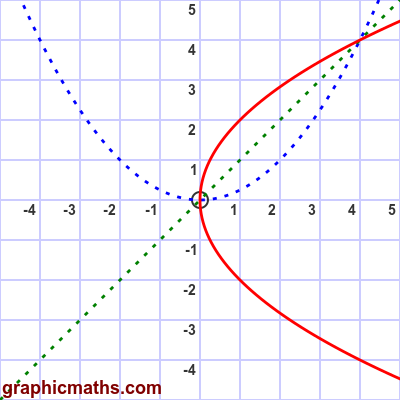# Cartesian equation of a parabola

By Martin McBride, 2020-09-12
Tags: parabola cartesian equation
Categories: coordinate systems pure mathematicsWe can convert the parametric equation of a parabola into a Cartesian equation (one involving only $x$ and $y$ but not $t$). Here are the parametric equations:

\begin{align} x = a t^2\newline y = 2 a t \end{align}

We can eliminate $t$ from these equations by first finding $t$ as a function of $y$:

\begin{align} y = 2 a t\newline t = \frac{y}{2 a} \end{align}

Then we can substitute $t$ in the equation for $x$

\begin{align} x &= a t^2\newline x &= a (\frac{y}{2 a})^2\newline x &= \frac{a y^2}{4 a^2}\newline x &= \frac{y^2}{4 a} \end{align}

This can also be written as:

$$y^2 = 4 a x$$

## A parabola is a quadratic curve

As you can see, a parabola involves a term in $y^2$ which means it is a quadratic curve. It has the classic quadratic curve shape. But because $x$ is a function of $y^2$, the x-axis and y-axis. swap roles. More accurately, the curve is mirrored about the line $y = x$.

In the graph, the red curve is our parabola (for $a = 1$), and for comparison the blue curve is the quadratic curve $y = x^2/4$. The green line is the line $y = x$.